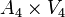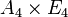# Direct product of A4 and V4

View a complete list of particular groups (this is a very huge list!)[SHOW MORE]

## Definition

This group is defined as the external direct product of the alternating group of degree four$A_4$ and the Klein four-group$V_4$ (the elementary abelian group of order 4). The group is denoted$A_4 \times V_4$ or$A_4 \times E_4$.

## GAP implementation

### Group ID

This finite group has order 48 and has ID 49 among the groups of order 48 in GAP's SmallGroup library. For context, there are groups of order 48. It can thus be defined using GAP's SmallGroup function as:

SmallGroup(48,49)

For instance, we can use the following assignment in GAP to create the group and name it$G$:

gap> G := SmallGroup(48,49);

Conversely, to check whether a given group$G$ is in fact the group we want, we can use GAP's IdGroup function:

IdGroup(G) = [48,49]

or just do:

IdGroup(G)

to have GAP output the group ID, that we can then compare to what we want.

### Other descriptions

Description Functions used
DirectProduct(AlternatingGroup(4),ElementaryAbelianGroup(4)) DirectProduct, AlternatingGroup, ElementaryAbelianGroup
DirectProduct(AlternatingGroup(4),CyclicGroup(2),CyclicGroup(2)) DirectProduct, AlternatingGroup, CyclicGroup